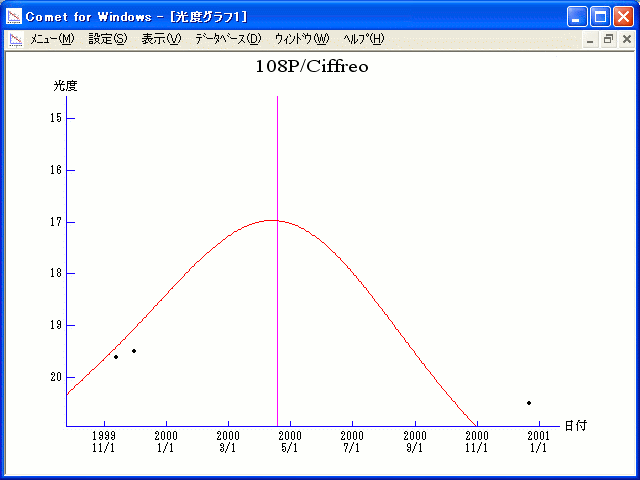# \$B%7%U%l%*WB@1(B

108P/Ciffreo (2000)###\$B50F;MWAG(B

```   The following improved orbital elements by Kenji Muraoka, are
from 102 observations  1985 to 1999, including 9 Planets, Moon
and 5 minor planets perturbations and non-gravitational effect
of style II.    The mean residual is +/- 0.93 arc seconds.

Epoch  =  2000 Apr.  6.0  TT       JDT = 2451640.5
T  =  2000 Apr. 18.39097       +/- 0.00230 (m.e.) TT
Peri. =  358.04249                +/- 0.00128
Node  =   53.72152                +/- 0.00007   (2000.0)
Incl. =   13.09288                +/- 0.00004
q  =    1.7133555              +/- 0.0000025 AU
e  =    0.5426166              +/- 0.0000029
a  =    3.7459937              +/- 0.0000066 AU
n  =    0.13594195             +/- 0.00000036
P  =    7.250                  +/- 0.0000192  years
A1  =   +0.243                  +/- 0.367
A2  =   -0.02479                +/- 0.00364
```

###\$B@1?^(B###\$B8wEYJQ2=(B

```        m1 = 9.0 + 5 log\$B&\$(B + 25.0 log r
```##### \$B50F;MWAG\$OB<2,7r<#;a\$N7W;;\$K\$h\$k\$b\$N\$G\$9!#(B \$B@1?^\$O(B StellaNavigator Ver.2.0 for Windows (\$B%"%9%H%m%"!<%D(B \$BJTCx(B / \$B%"%9%-!<=PHG6I4)(B) \$B\$G:n@.\$7\$?\$b\$N\$G\$9!#(B \$B8wEY%0%i%U\$O(BComet for Windows\$B\$G:n@.\$7\$?\$b\$N\$G\$9!#(B